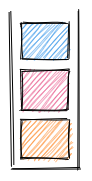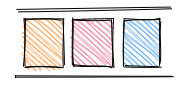Stacks and Queues

# Stacks and Queues

2020-02-17T23:46:37.187Z

# Stacks

A stack is container where pieces of data enter and exit from one end, following the last-in-first-out (LIFO) principle.

A stack stores data just like arrays do—a stack is simply a list of elements, but with the following constraints

• Data can only be inserted at the end of a stack.
• Data can only be read from the end of a stack.
• Data can only be removed from the end of a stack.

Adding to a stack is called "push" and removing from the stack is called "popping". The end of the stack is called its "top" and and the beginning of the stack is called its "bottom".

Stacks are often used for implementing an undo feature or navigation. Stacks are similar to queues except for the order in which the items come out.Stack

Stacks are not used for lookup.

Stacks are useful for reversing or doing something in reverse order.

All operations in a stack run in `O(1)`.

Operation Runtime
push (add item on top) `O(1)`
pop (remove item on top) `O(1)`
peek (show item on top) `O(1)`
empty `O(1)`

Stacks can be implemented using arrays or linked lists.

``````public class Main {
public static void main(String[] args) {
Stack<Integer> stack = new Stack<>();
stack.push(10);
stack.push(20);
stack.push(30);
}
}
``````

## Common operations

Reversing a string with `String`:

``````public String reverse(String input) {

Stack<Character> stack = new Stack<>();
for (i = 0; i < input.length(); i++) {
Stack.push(input.charAt(i))
}

String result;
while (!stack.empty()) {
result += stack.pop();
}
return result;
}
``````

Reversing a string with `StringBuffer`:

``````public String reverse(String input) {

Stack<Character> stack = new Stack<>();
for (i = 0; i < input.length(); i++) {
Stack.push(input.charAt(i))
}

StringBuffer result = new StringBuffer();
while (!stack.empty()) {
result.append(stack.pop());
}
return result.toString();
}
``````

Going backward to check if a closing bracket has a matching opening bracket:

``````public boolean isBalanced(String input)  {
Stack<Character> stack = new Stack<>();
for (char ch : input.toCharArray()) {
if (ch == "(")
stack.push(ch);
if (ch == ")") {
if (stack.empty()) return false; // for ")1 + 2("
stack.pop();
}
}
return stack.empty();
}
``````

## Implementation

Implementing a stack in Java:

``````public class Stack {
private int[] items = new int;
private int[] count;

public void push(int item) {
if (count == items.length()) {
throw new StackOverflowError();
}

items[count] = item;
count++;
}

public int pop() {
if (count == 0)
throw new IllegalStateException();
count--;
return items[count];
}

public int peek() {
if (count == 0) throw new IllegalStateException();
return items[count - 1];
}

@Override
public String toString() {
var content = Arrays.copyOfRange(items, 0, count);
return Arrays.toString(content);
}
}
``````

Implementing a stack in JavaScript:

``````class Queue {
constructor() {
this.data = [];
}
push(item) {
this.data.push(item);
}
pop() {
return this.data.pop();
}
peek() {
return this.data[this.data.length - 1];
}
}
``````

# Queues

A queue is a container where pieces of data enter on one end and exit on the opposite end. Stacks follow the first-in-first-out (FIFO) principle: The first item to come into the queue is the first item to come out of the queue.

Queues are arrays with three restrictions:

• Data can only be inserted at the back of a queue, as in the stack.
• Data can only be read from the front of a queue.
• Data can only be removed from the front of the queue.

Adding to a queue is called "enqueuing" and removing from the queue is called "dequeuing". Showing the last item in the queue is called "peeking". The first item in the queue is called the "front" and the last item in the queue is called the "back".Queue

Queues are used for processing jobs in the order they are received in.

Operation Runtime
enqueue (add item to back of queue) `O(1)`
dequeue (add item to front of queue) `O(1)`
peek (show item at back of queue) `O(1)`
empty `O(1)`
isFull `O(1)`
enqueue (in priority queue) `O(n)`

In Java, a `Queue` is an interface, not a class, and therefore cannot be instantiated.

An `ArrayDeque` is a double-ended queue.

``````public class Main {
public static void main(String[] args) {
Queue<Integer> queue = new ArrayDeque<>();
var front = queue.remove(); // → 10
}
}
``````

## Common operations

To reverse a queue, place its items in a stack and pop them out one by one back into the queue.

``````public static void reverse(Queue<Integer> queue) {
Stack<Integer> stack = new Stack<>();
while (!queue.isEmpty) {
stack.push(queue.remove());
}
while (!stack.isEmpty) {
}
}
``````

## Implementation

Implementing a queue using an array in Java:

``````public class ArrayQueue {
private int[] items;
private int rear;
private int count;
private int front;

public ArrayQueue(int capacity) {
items = new int[capacity];
}

public void enqueue(int item) {
if (count == items.length)
throw new IllegalStateException();
items[rear] = item;
rear = (rear + 1) % items.length; // circular array
count++;
}

public int dequeue() {
var item = items[front];
items[front] = 0;
front = (front + 1) % items.length; // circular array
count--;
return item;
}

@Override
public String toString() {
return Arrays.toString(items);
}
}
``````

Implementing a queue using two stacks in Java:

``````public class QueueWithTwoStacks {
private Stack<Integer> stack1 = new Stack<>();
private Stack<Integer> stack2 = new Stack<>();

public void enqueue(int item) {
stack1.push(item);
}

public int dequeue() {

if (isEmpty())
throw new IllegalStateException();

moveStack1ToStack2();
return stack2.pop();
}

public int peek() {
if (isEmpty())
throw new IllegalStateException();
moveStack1ToStack2();
return stack2.peek();

}

public boolean isEmpty() {
return stack1.isEmpty() && stack2.isEmpty();
}

private void moveStack1ToStack2() {
if (stack2.isEmpty())
while (!stack1.isEmpty())
stack2.push(stack1.pop());
}
}
``````

Queues as implemented in JavaScript:

In JavaScript, there is no such thing as a rudimentary queue, because a JavaScript array encompasses what a queue does and much more.

Taking an array and restricting the methods that can be used to interact with that array. In other words, you are "handicapping" the array.

``````class Queue {
constructor() {
this.data = [];
}
enqueue(item) {
this.data.unshift(item);
}
dequeue() {
return this.data.pop();
}
peek() {
return this.data[this.data.length - 1];
}
}
``````

You can also implement a queue using two stacks: the first stack receives all the items and pops them back out and into the second stack, so that the second stack can pop them back out in the order they were originally pushed in.

Priority queue:

``````public class Main {
public static void main(String[] args) {
PriorityQueue<Integer> queue = new PriorityQueue<>();
queue.remove(); // removes lowest number first
}
}
``````

Implementing a priority queue:

``````public class PriorityQueue {
private int[] items = new int;
private int count; // initialized to 0

public void add(int item) {
if (count == items.length)
throw new IllegalStateException();

int i;
for (i = count - 1; i >= 0; i--) {
if (items[i] > item)
items[i + 1] = items[i]; // shift items forward...
else
break;
}
items[i + 1] = item; // ...in order to insert this item
count++;
}

public int remove() {
if (count == 0)
throw new IllegalStateException();

count--;
return items[--count];
}

@Override
public String toString() {
return Arrays.toString(items);
}
}
``````